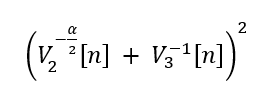# How to express this formula by CVX

Hello, everyone !
`V2` and `V3` are both variables, how to write the expression by `CVX` ?Make use of `square_pos` and `pow_p` provided by CVX, you will work it out.

Is it express in this way ?

square_pos( pow_p(V2(n),-alpha/2)+ pow_p(V3(n),-1) )

Yes.

But for this to be accepted by CVX, `alpha` must be nonnegative.

In this formulation, CVX (`pow_p` and `inv_pos`) will implicitly constrain `V2(n)` and `V3(n)` to be nonnegative.

OK, Thank you very much !